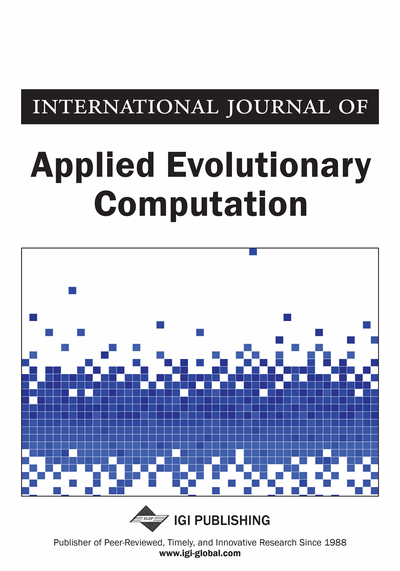# An Incorporation of the Fuzzy Greedy Search Heuristic With Evolutionary Approaches for Combinatorial Optimization in Operations Management

Kaveh Sheibani
DOI: 10.4018/IJAEC.2017040104
OnDemand:
(Individual Articles)
Available
\$37.50
No Current Special Offers

## Abstract

Although greedy algorithms are important, nowadays it is well assumed that the solutions they obtain can be used as a starting point for more sophisticated methods. This paper describes an evolutionary approach which is based on genetic algorithms (GA). A constructive heuristic, so-called fuzzy greedy search (FGS) is employed to generate an initial population for the proposed GA. The effectiveness and efficiency of the proposed hybrid method are demonstrated on permutation flow-shop scheduling as one of the most widely studied hard combinatorial optimization problems in the area of operational research.
Article Preview
Top

## Introduction

In recent decades, there has been a growth of interest in methods for finding optimal solutions to a class of problems called combinatorial optimization. The subject is very wide and many books and articles have been published on its various aspects. Typical examples of combinatorial optimization problems are the travelling salesman (Lawler, 1985; Gutin and Punnen, 2002; Gutin, 2013), variants of the assignment problem (Cela et al., 2014), scheduling problems (Pinedo et al., 2015), the set covering (Beasley, 1990; Boschetti and Maniezzo, 2015), and vehicle routing problems (Coelho and Laporte, 2015). Combinatorial problems are normally easy to describe but difficult to solve (Korte and Vygen, 2012). The foundations for the theory of computational complexity are to be found in Cook’s (1971) seminal paper. In his paper, Cook attempted to classify problems in practice as easy or hard. A problem is called easy if we can develop an algorithm which solves the problem to optimality in a polynomial-time (Du and Pardalos, 2005). A problem is called hard or intractable if such efficient algorithms do not exist or the solution cannot be found within a reasonable computational time bound.

Due to the practical importance of combinatorial optimization problems, many methods have been developed for solving them (Pulleyblank, 2014); Martello and Ries, 2015); Mohanty et al., 2017). These methods can be classified as either exact or approximate. Exact methods guarantee to find an optimal solution in a bounded amount of time. Of course, for those combinatorial optimization problems which belong to the class NP-hard (Garey and Johnson, 1979), exact methods need an exponential amount of time. Thus, approximation methods which usually called heuristics, are often considered to be the only practical tools available to solve hard combinatorial optimization problems.

This paper introduces a new idea for the integration of approaches for hard combinatorial optimization problems. The paper consists of two main parts. The first part focuses on description of the theory and mathematics of the so-called fuzzy greedy evaluation concept. The second part demonstrates computational examples of the proposed concept for hard combinatorial optimization problems. The concluding remarks contain some suggestions for further research.

### Fuzzy Sets

In many real-world problems fuzzy sets allow us to represent vague concepts expressed in natural language. The membership function of a fuzzy set A can be denoted by µA: X → [0,1]. Each fuzzy set should be uniquely defined by one particular membership function. Consider a fuzzy set where membership function is defined in Equation (1). This is one of the general formulae of a parameterized family of membership functions described in Klir and Yuan (1995).

(1)

This fuzzy set expresses, in a particular form, the general concept of a class of real numbers that are close to r. When the non-negative parameter ρ increases, the graph of µA(x) becomes narrower. The function has the following properties: µA(r) = 1 and µA(x) < 1 for all xr. For a complete discussion of fuzzy sets, we refer to (Klir and Yuan, 1995; Zimmermann, 2001; Wang and Klir, 2013).

## Complete Article List

Search this Journal:
Reset
Volume 14: 1 Issue (2023): Forthcoming, Available for Pre-Order
Volume 13: 4 Issues (2022): 2 Released, 2 Forthcoming
Volume 12: 4 Issues (2021)
Volume 11: 4 Issues (2020)
Volume 10: 4 Issues (2019)
Volume 9: 4 Issues (2018)
Volume 8: 4 Issues (2017)
Volume 7: 4 Issues (2016)
Volume 6: 4 Issues (2015)
Volume 5: 4 Issues (2014)
Volume 4: 4 Issues (2013)
Volume 3: 4 Issues (2012)
Volume 2: 4 Issues (2011)
Volume 1: 4 Issues (2010)
View Complete Journal Contents Listing